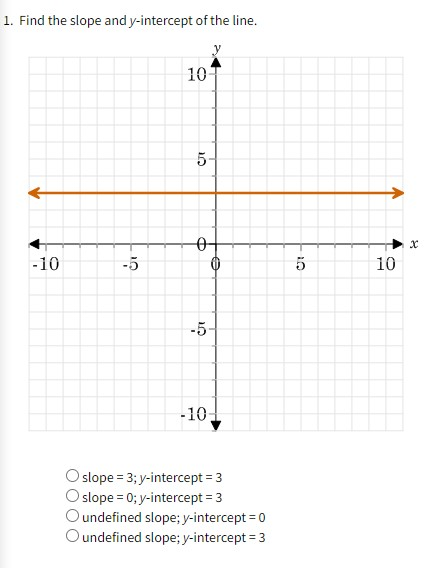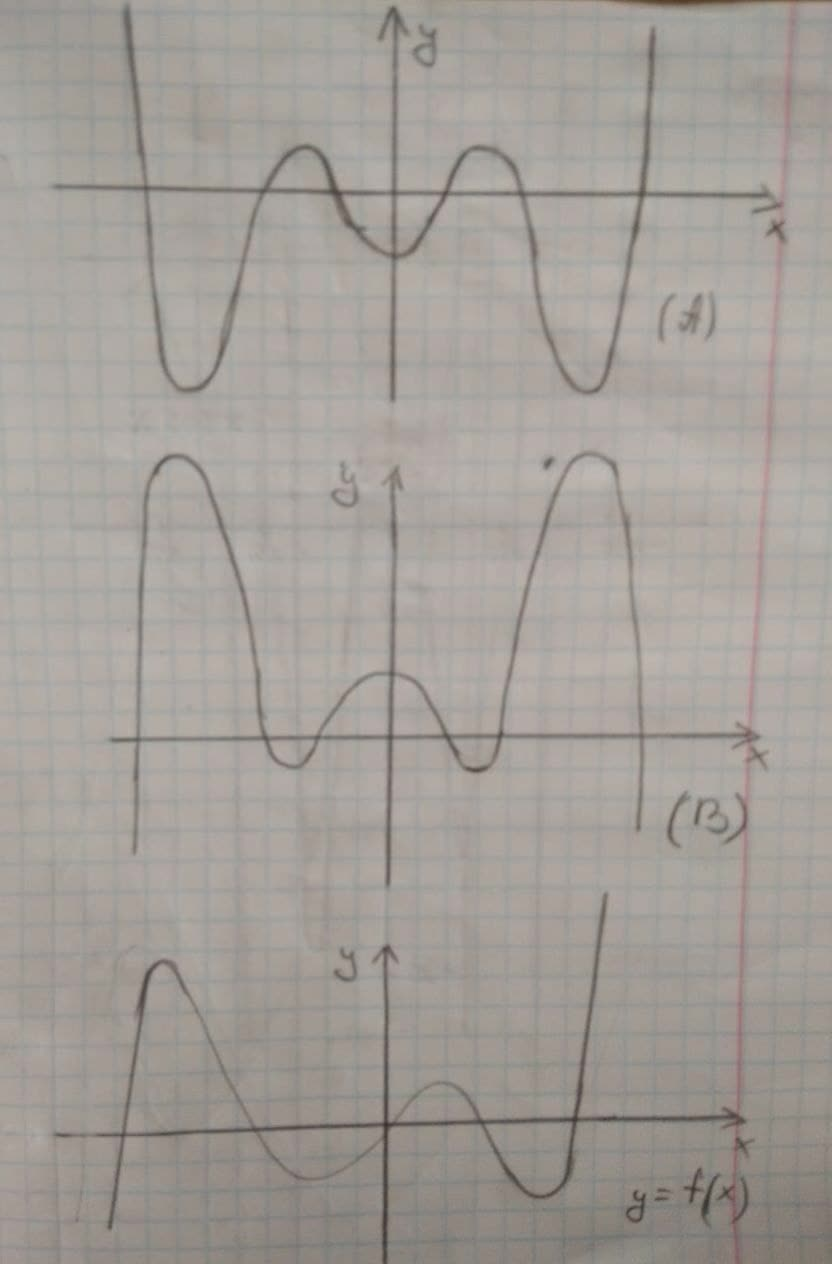# Polynomial Graphs Examples: See the Power of Polynomials in Action

Recent questions in Polynomial graphsnepojamanuszc 2023-03-26

## Determine the value of k if the remainder is 3 given $\left({x}^{3}+k{x}^{2}+x+5\right)÷\left(x+2\right)$gelepikb165 2023-02-22

## Factor completely ${x}^{3}-2{x}^{2}-9x+18$Jaslyn Gordon 2023-02-21

## use the remainder theorem to find the remainder for the division $\left(3{t}^{3}-10{t}^{2}+t-5\right)÷\left(t-4\right)$Clinton Mccormick 2023-02-16

## Determine the binomial factors of ${x}^{3}+3{x}^{2}+3x+1$Jaycee Cox 2023-02-14

## Use the remainder theorem to find P(c) $P\left(x\right)=6{x}^{3}-{x}^{2}+4x+3$, a=3.l1voyax 2023-02-10

## Use synthetic division and the Remainder Theorem to find the indicated function value $f\left(x\right)=2{x}^{3}-11{x}^{2}+7x-5$; f(4).Horlando Montiel Antonio [STUDENT]2022-08-25

## m=- 3/4, b=2

Algebra IIOpen questionkaidance grubbs richard2022-08-24

##Algebra IIOpen questionkaidance grubbs richard2022-08-24

##Filipinacws 2022-08-08

## Determine whether the function is even, odd, or neither. Then determine whether the function's graph is symmetric with respect to the y-axis, the origin, or neither. $h\left(x\right)=3{x}^{4}-{x}^{6}$Determine whether the function is even, odd, or neither. Choose the correct answer below. a. odd b. even c. neither

Algebra IIOpen questionISAIAH THOMAS2022-05-26

## Find a polynomialf(x)of degree4that has the following zeros. 2, -8, -6, 7Njd gang2022-04-06

## come up with a rational function that has four asymptotes and three x intercepts.dictetzqh 2021-12-05

## Evaluate the integral to find the area between the curves: $f\left(x\right)=x$ $g\left(x\right)={x}^{2}-2x$angepepagiortzb 2021-12-04

## In Figure 3, is graph (A) or graph (B) the graph of an antiderivative of $y=f\left(x\right)$?enfurezca3x 2021-12-03

## Write an equation for a rational function with: Vertical asymptotes at x intercepts at Horizontal asymptote at $y=8$Michelle Isakson 2021-12-03

## Graph in the same viewing rectangle.skomminbv 2021-11-30

## Use the intermediate value theorem to determine whether the function $f\left(x\right)={x}^{3}+2x-4$ has a root or not. between . Ifyes, then find the root to five decimal places.Yasmin 2021-08-21Chardonnay Felix 2021-08-18